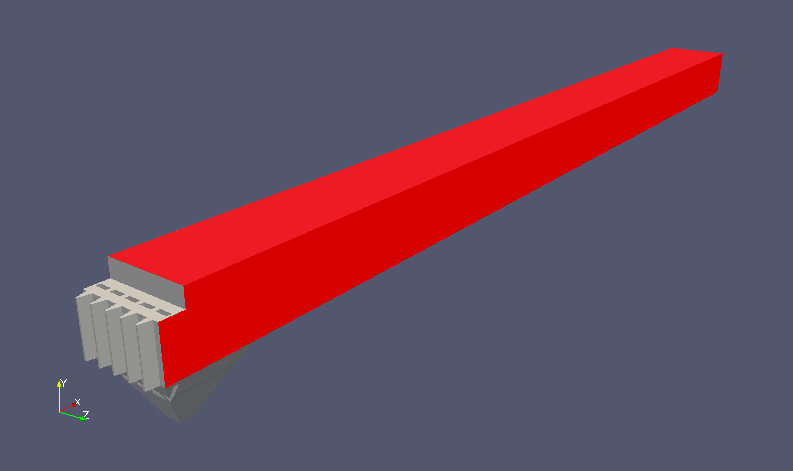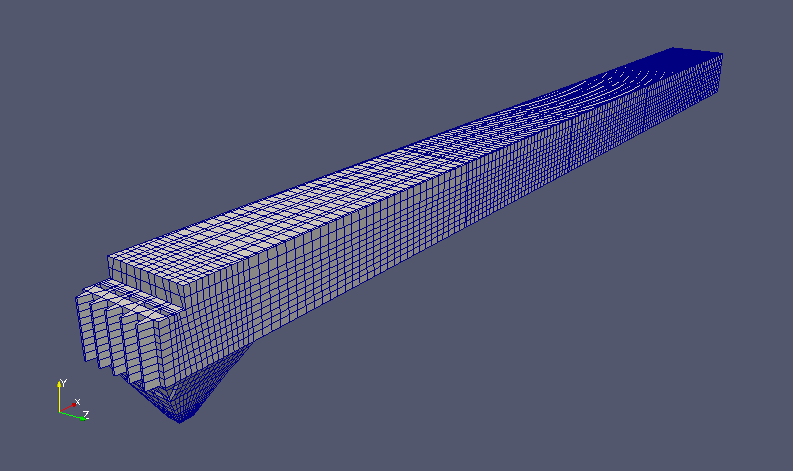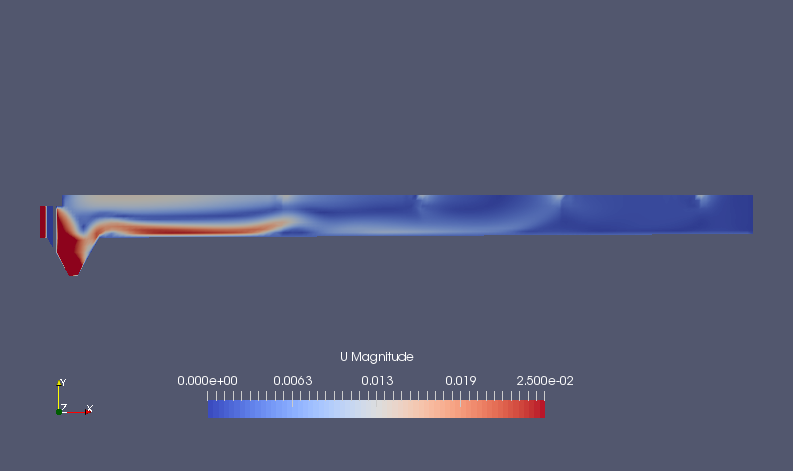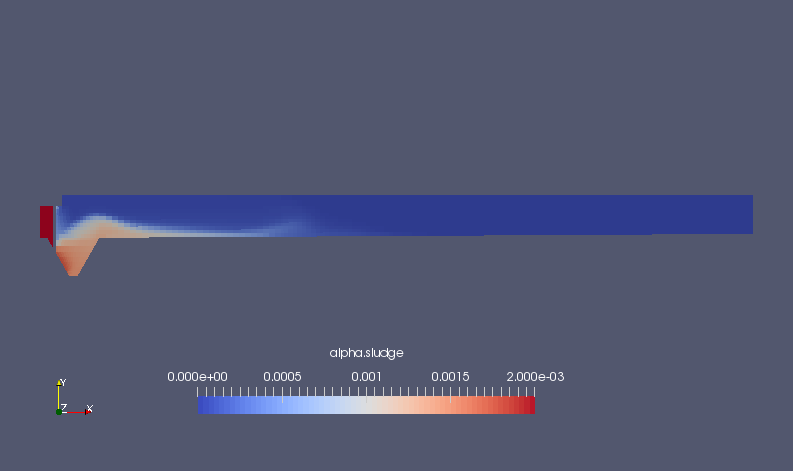﻿ Inflow and outflow of muddy water into the tank - XSim

# Inflow and outflow of muddy water into the tank

OpenFOAM 4.x

## Case directory

\$FOAM_TUTORIALS/multiphase/driftFluxFoam/ras/tank3D

## Summary

We calculate the inflow/outflow of muddy water into a tank filled with water for 8000 seconds. Assume that water with a volume fraction of 0.2% sludge flows in at (0.1315, 0, 0) m/s from region "INLE1" and out at (0 0.0177 0) m/s from regions "OUTL9", "OUTL10", "OUTL11", and "OUTL12". Region "OUTL15" is assumed to be the inlet and outlet.

In addition, region "WALL6" is assumed to be a wall moving at (-0.003, 0, 0) m/s, region "SYMP3" is assumed to be a slip wall, and the other walls are assumed to be no-slip walls.Model geometryRegion SYMP3 (red part)

The properties of the sludge and water are specified in the file "constant/transportProperties" as follows.

```phases (sludge water);

sludge
{
transportModel  BinghamPlastic;

"(plastic|BinghamPlastic)Coeffs"
{
coeff       0.00023143;
exponent    0.17926;

BinghamCoeff    7.845e-07;
BinghamExponent 95.25;
BinghamOffset   0;

muMax       10;
}

rho         1042;
}

water
{
transportModel  Newtonian;

nu              1.78e-06;
rho             1000;
}

relativeVelocityModel simple;

"(simple|general)Coeffs"
{
V0              (0 -0.002198 0);
a               8.84;
a1              0.1;
residualAlpha   0;
}

```

The meshes are as follows, and the number of mesh is 19166.Meshes

The calculation result is as follows.

Volume ratio of sludge (alpha.sludge)Flow velocity at final timeVolume ratio of sludge at final time (alpha.sludge)

## Commands

cp -r \$FOAM_TUTORIALS/multiphase/driftFluxFoam/ras/tank3D tank3D
cd tank3D

driftFluxFoam

paraFoam

## Calculation time

40 minutes 59.6 seconds *Single, Inter(R) Core(TM) i7-2600 CPU @ 3.40GHz 3.40GHz